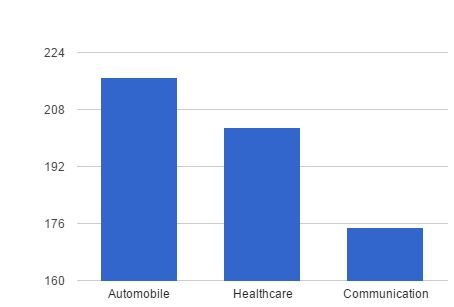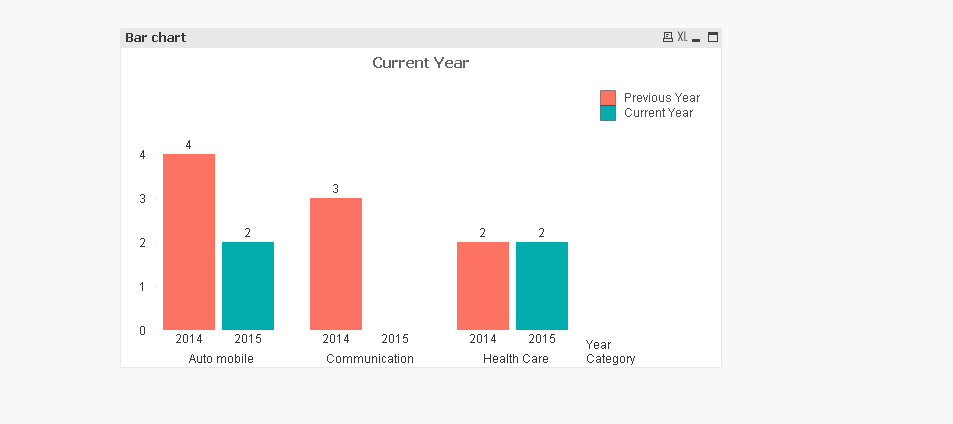# New to QlikView

Discussion board where members can get started with QlikView.

Announcements
BARC’s The BI Survey 19 makes it official. BI users love Qlik. GET REPORT
Highlighted
Contributor III

I need to show Number of Products sold each year for different Categories.

Something like this• Here Category will be the Primary Dimension and Year will be the Secondary Dimension.
• count(Products Sold) will be the expression.

However, I need to show the count only for Current and Previous Year. Current Year data will be updated monthly. For that purpose there is a field called UpdatedDate.

Assume, Current Year is 2015 and Previous Year is 2014. 2014 data will be same while 2015 data changes every month. I need to the bar chart accordingly.

I tried this as a Calculated Dimension

If(

Year=(max(Year)-1), Year,

if(

Year=max(Year) and UpdatedDate=max(UpdatedDate),Year

)

)

This is not working. I know I'm wrong logically somewhere. Can someone help me on how to solve this?

Tags (3)
1 Solution

Accepted SolutionsMVP

Set analysis would be a better way to achieve this. Having said that, if you want to know what went wrong the way you tried and get it corrected, try like:

If(

Year=\$(=max(Year)-1), Year,

if(

Year=\$(=max(Year)) and UpdatedDate=\$(=max(UpdatedDate)),Year

)

)

13 RepliesMVP

Current :

=count({<Year ={"\$(=max(Year))"}>}Products Sold)

Previous:

=count({<Year ={"\$(=max(Year)-1)"}>}Products Sold)MVP

Try,

Aggr(Only({<Year={'\$(=Max(Year))','\$(=Max(Year)-1)'}>} Year),Year)

Honored Contributor

Hi,

You can use two expression for comparison it will be easy ,

Dimension :

Category

Expressions:

Previous Year

Count({< Year={\$(=Max(Year)-1)}>}Products Sold)

Current year

Count({< Year={\$(=Max(Year))}>}Products Sold)

Post your sample data if possbile.

HTH,

-Hirish

HirisH
“Aspire to Inspire before we Expire!”
Contributor III

Hi Tamil,

This will work perfectly. But I need to include the max(UpdatedDate) for the Current Year since there is new data every month. Is there a way to do that?MVP

Set analysis would be a better way to achieve this. Having said that, if you want to know what went wrong the way you tried and get it corrected, try like:

If(

Year=\$(=max(Year)-1), Year,

if(

Year=\$(=max(Year)) and UpdatedDate=\$(=max(UpdatedDate)),Year

)

)

Contributor III

Hi Harish and Kush141087‌,

I tried this using two expressions. I guess I cannot make use of Legend selection. Correct me if I'm wrong.MVP

Fine.

=Aggr(Only({<Year={'\$(=Max(Year)-1)'}>+<Year={'\$(=Max(Year))'},UpdatedDate={'\$(=Date(Max(UpdatedDate),"DD/MM/YYYY"))'}>} Year),Year)

Change the date format accordingly.

Honored Contributor

Hi,

Check this App with sample data,At Back end:

```Data:
[Updated Date],
Month([Updated Date]) as Month,
Year([Updated Date]) as Year,
[Products Sold]
FROM
[\Dataforprecurr.xlsx]
(ooxml, embedded labels, table is Sheet1);

```

At Front end:

In Chart:

Dimensions:

Category and Year,

Expressions:

Previous and current year expressions

HTH ,

-Hirish.

HirisH
“Aspire to Inspire before we Expire!”
Valued Contributor III